Informally Parameters and properties of DC Motors Operating

• Slides: 44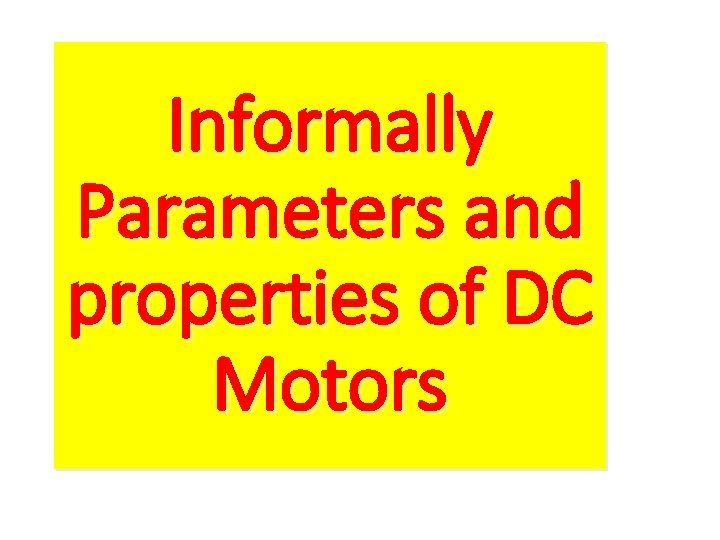Informally Parameters and properties of DC Motors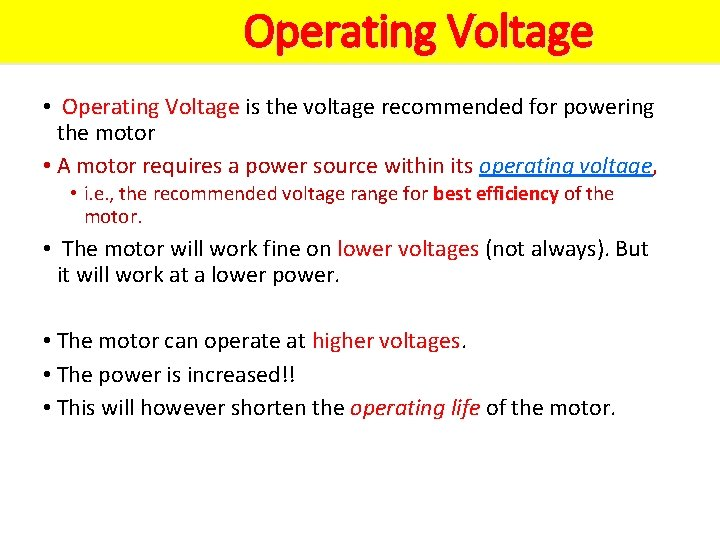Operating Voltage • Operating Voltage is the voltage recommended for powering the motor • A motor requires a power source within its operating voltage, • i. e. , the recommended voltage range for best efficiency of the motor. • The motor will work fine on lower voltages (not always). But it will work at a lower power. • The motor can operate at higher voltages. • The power is increased!! • This will however shorten the operating life of the motor.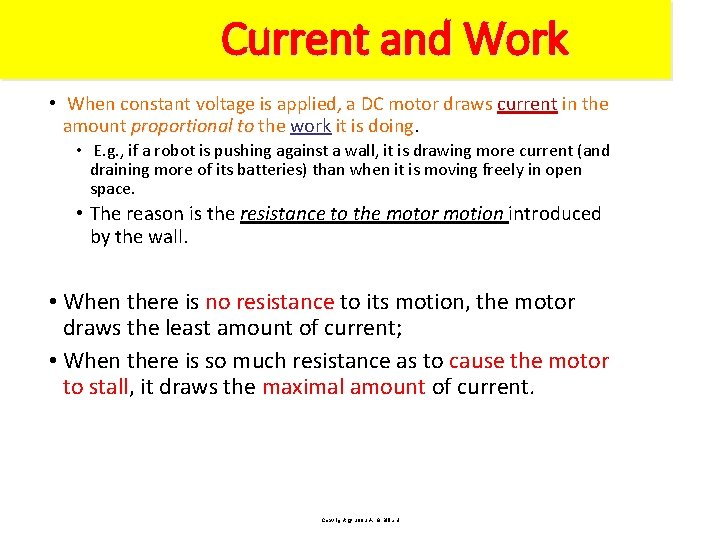Current and Work • When constant voltage is applied, a DC motor draws current in the amount proportional to the work it is doing. • E. g. , if a robot is pushing against a wall, it is drawing more current (and draining more of its batteries) than when it is moving freely in open space. • The reason is the resistance to the motor motion introduced by the wall. • When there is no resistance to its motion, the motor draws the least amount of current; • When there is so much resistance as to cause the motor to stall, it draws the maximal amount of current. Copyright @ 2002 A. G. Billard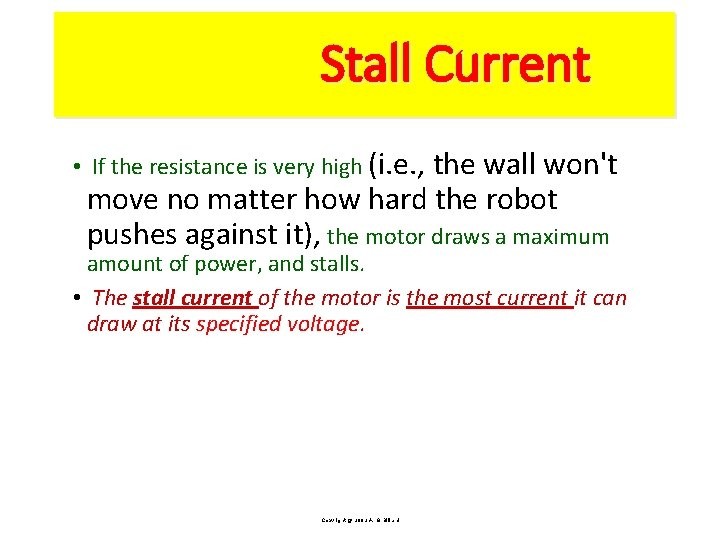Stall Current • If the resistance is very high (i. e. , the wall won't move no matter how hard the robot pushes against it), the motor draws a maximum amount of power, and stalls. • The stall current of the motor is the most current it can draw at its specified voltage. Copyright @ 2002 A. G. BillardMotor Inefficiency • As any physical system, DC motors are not perfectly efficient. • The energy is not converted perfectly. • Some energy is wasted as heat generated by friction of mechanical parts. • Inefficiencies are minimized in well-designed (more expensive) motors, and their efficiency can be high. • How high? Copyright @ 2002 A. G. Billard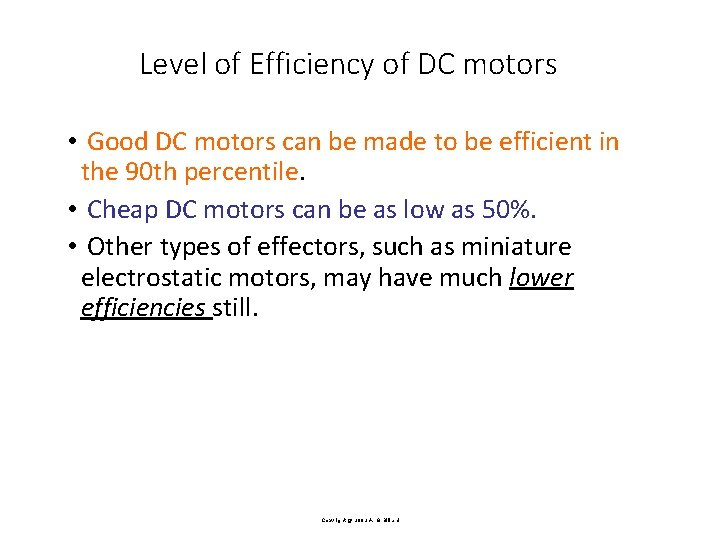Level of Efficiency of DC motors • Good DC motors can be made to be efficient in the 90 th percentile. • Cheap DC motors can be as low as 50%. • Other types of effectors, such as miniature electrostatic motors, may have much lower efficiencies still. Copyright @ 2002 A. G. Billard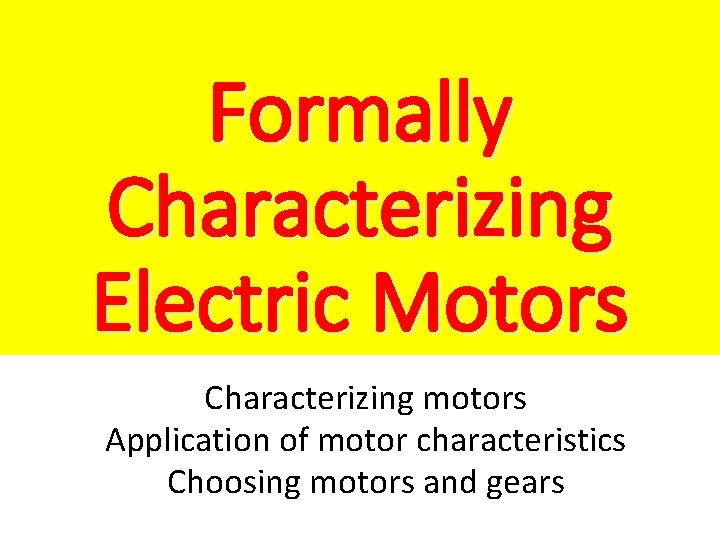Formally Characterizing Electric Motors Characterizing motors Application of motor characteristics Choosing motors and gearsCharacterizing Motors Power Torque Speed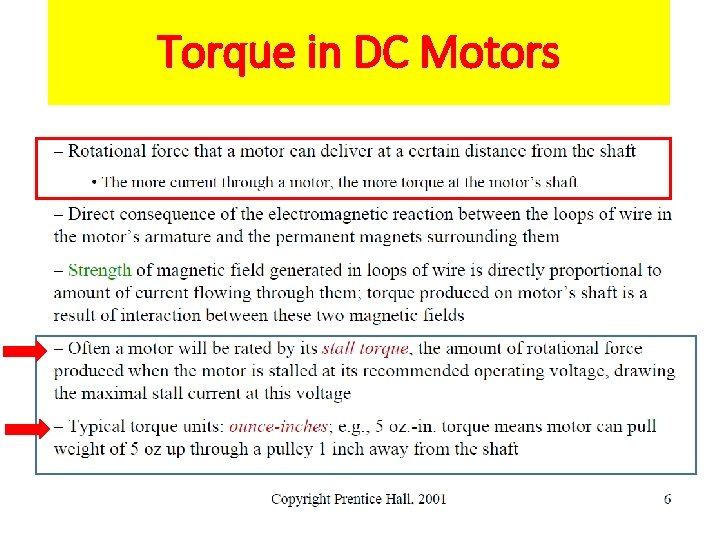Torque in DC Motors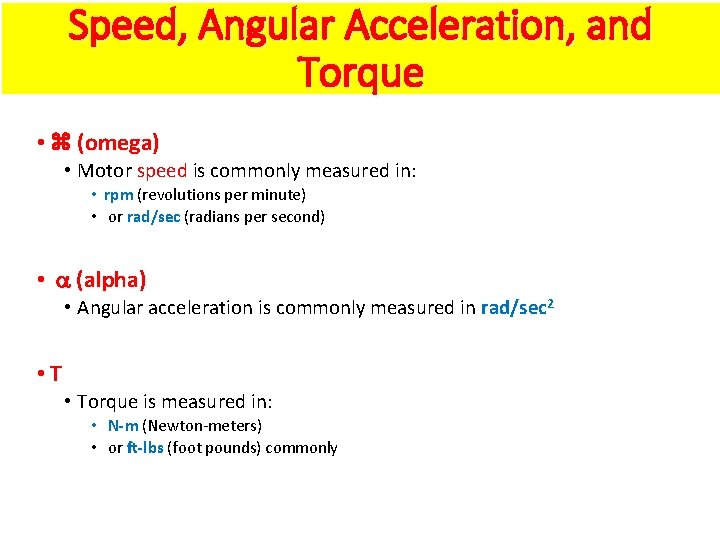Speed, Angular Acceleration, and Torque • (omega) • Motor speed is commonly measured in: • rpm (revolutions per minute) • or rad/sec (radians per second) • (alpha) • Angular acceleration is commonly measured in rad/sec 2 • Torque is measured in: • N-m (Newton-meters) • or ft-lbs (foot pounds) commonly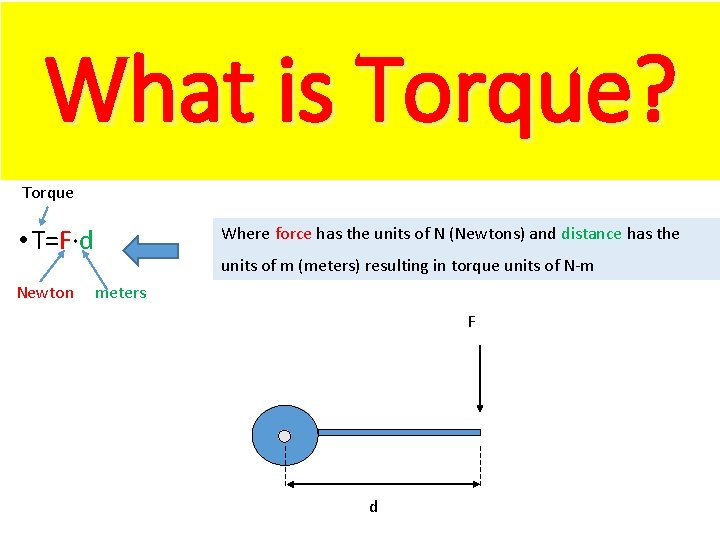What is Torque? Torque Where force has the units of N (Newtons) and distance has the • T=F·d Newton units of m (meters) resulting in torque units of N-m meters F dTorque at the Motor Shaft • Within a motor's operating current range, the more current is used, the more torque or rotational force is produced at the shaft. • The strengths of the magnetic field generated in the wire loops is directly proportional to the applied current and thus the produced torque at the shaft. Copyright @ 2002 A. G. Billard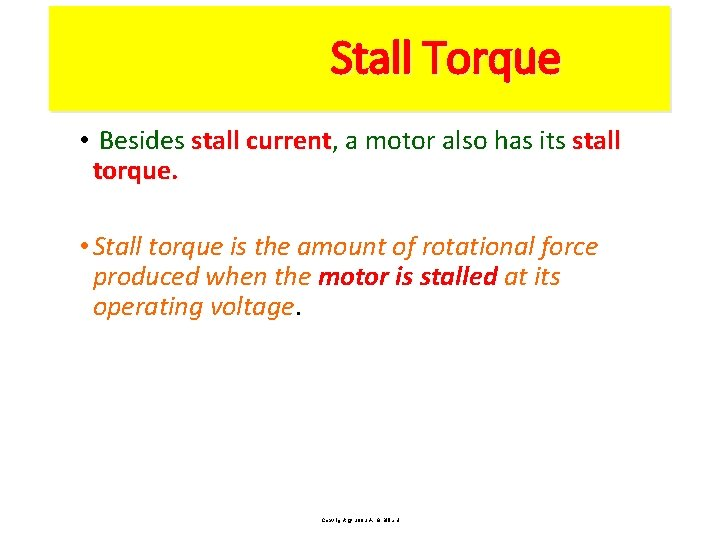Stall Torque • Besides stall current, current a motor also has its stall torque. • Stall torque is the amount of rotational force produced when the motor is stalled at its operating voltage. Copyright @ 2002 A. G. BillardFree Spinning and Stalling • When the motor is stalled, it is producing maximum torque, torque but the rotational velocity is 0, so the output power is 0 again. • Between free spinning and stalling, the motor does useful work, and the produced power has a characteristic parabolic relationship • A motor produces the most power in the middle of its performance range. No load, maximum speed but no power Copyright @ 2002 A. G. BillardPower Trends for Motors with linear torque speed curves Use such curves. provided by the producer, for your calculations SPEED • The amount of power a motor generates is the product of the shaft's rotational velocity and its torque. • If there is no load on the shaft, i. e. , the motor is spinning freely, then the rotational velocity is the highest • but the torque is 0, since nothing is being driven by the motor. • The output power, then, is also 0.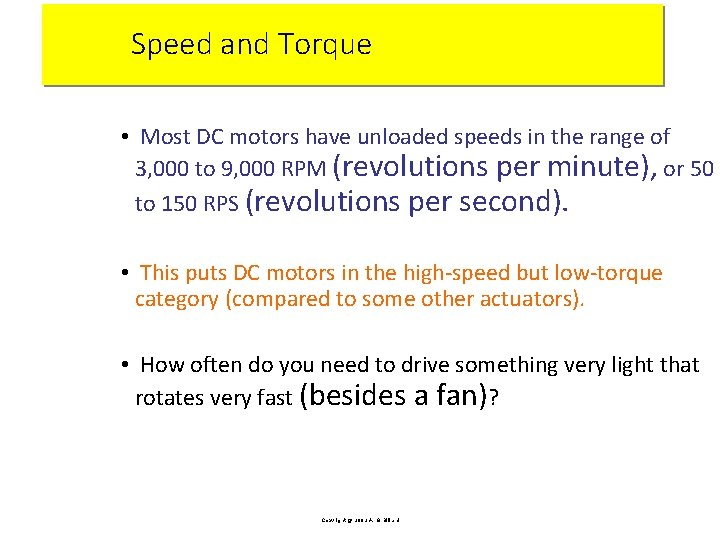Speed and Torque • Most DC motors have unloaded speeds in the range of 3, 000 to 9, 000 RPM (revolutions per minute), or 50 to 150 RPS (revolutions per second). • This puts DC motors in the high-speed but low-torque category (compared to some other actuators). • How often do you need to drive something very light that rotates very fast (besides a fan)? Copyright @ 2002 A. G. Billard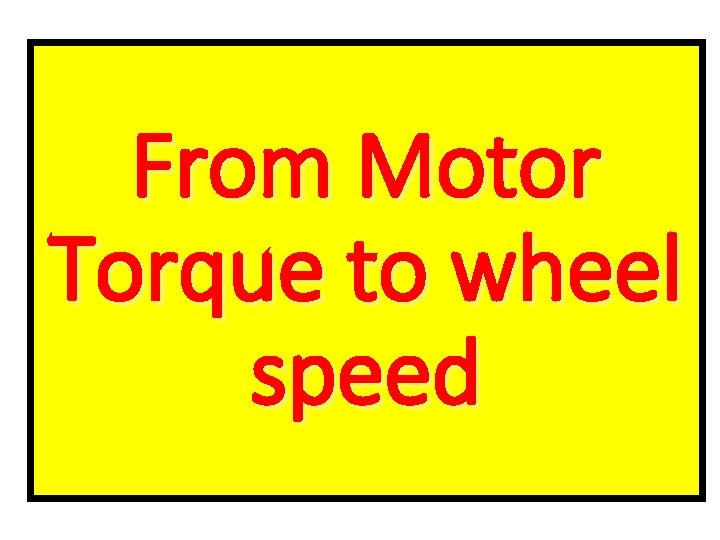From Motor Torque to wheel speed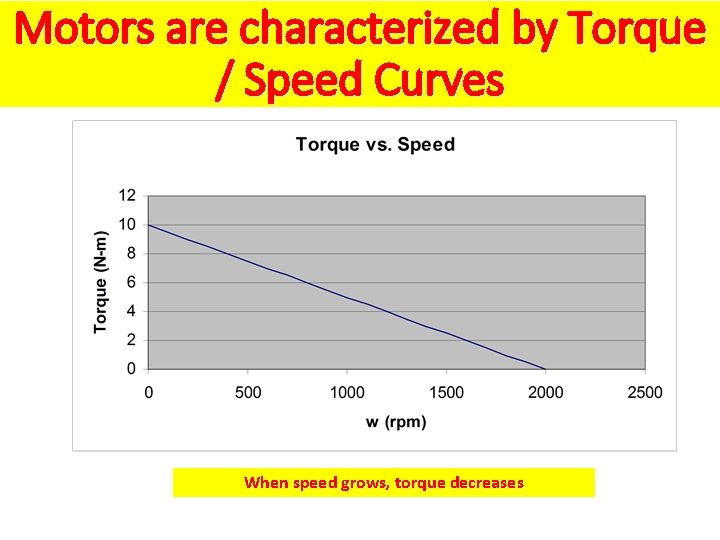Motors are characterized by Torque / Speed Curves When speed grows, torque decreases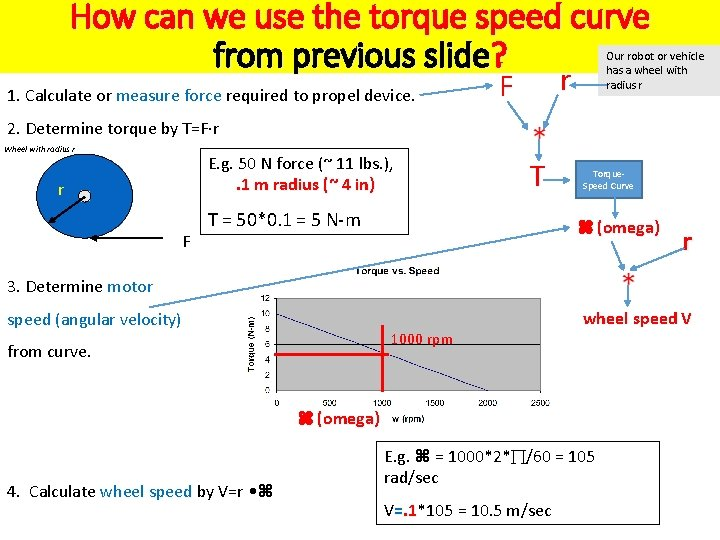How can we use the torque speed curve from previous slide? 1. Calculate or measure force required to propel device. Our robot or vehicle has a wheel with radius r r F 2. Determine torque by T=F·r Wheel with radius r E. g. 50 N force (~ 11 lbs. ), . 1 m radius (~ 4 in) r F T T = 50*0. 1 = 5 N-m Torque. Speed Curve (omega) r 3. Determine motor speed (angular velocity) 1000 rpm from curve. wheel speed V (omega) 4. Calculate wheel speed by V=r • E. g. = 1000*2* /60 = 105 rad/sec V=. 1*105 = 10. 5 m/sec. 1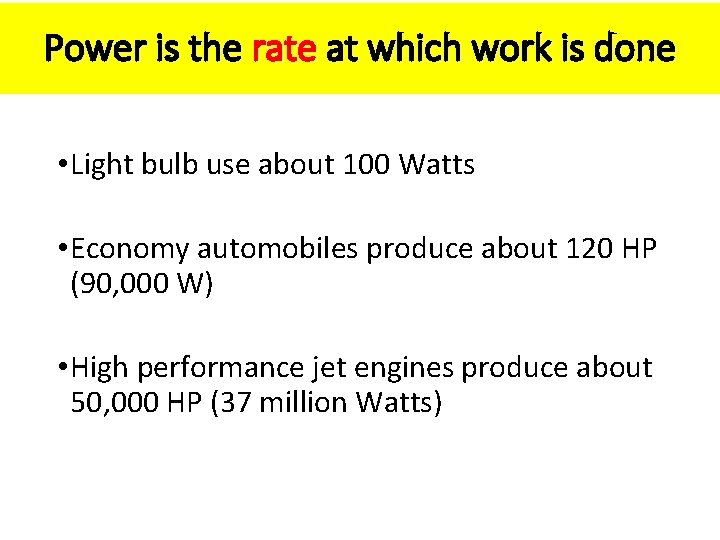Power is the rate at which work is done • Light bulb use about 100 Watts • Economy automobiles produce about 120 HP (90, 000 W) • High performance jet engines produce about 50, 000 HP (37 million Watts)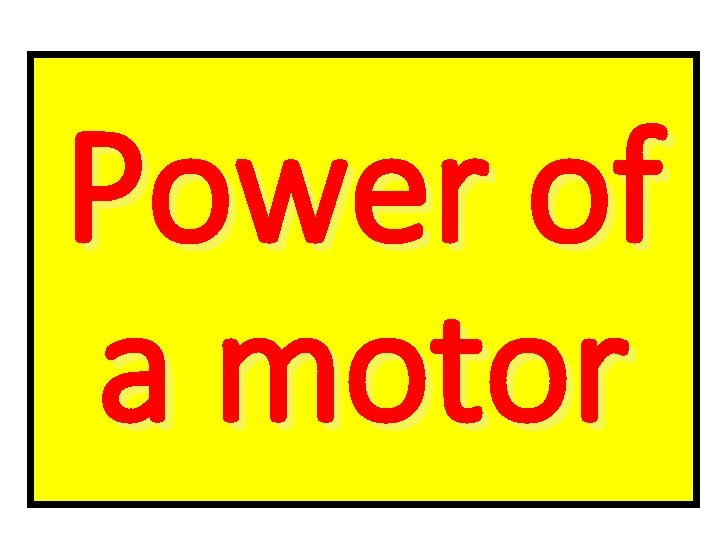Power of a motorPower of a motor • P=T • • Power is the torque times the angular velocity • If T is in N-m and is in rad/sec, power has the units of WattsPower in DC Motors • Think about a robot arm, you are trying to select the motors for shoulder. • How many motors? • How long the arm? • How many motors in the lower part of the arm? • How to transmit motion to fingers?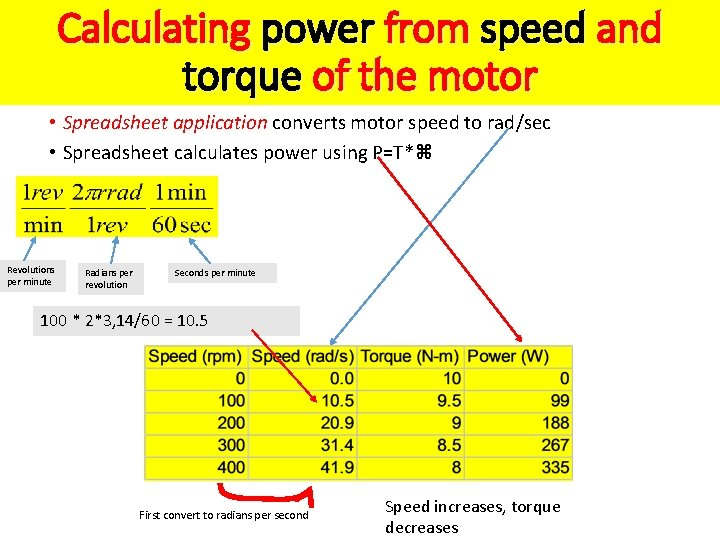Calculating power from speed and torque of the motor • Spreadsheet application converts motor speed to rad/sec • Spreadsheet calculates power using P=T* Revolutions per minute Radians per revolution Seconds per minute 100 * 2*3, 14/60 = 10. 5 First convert to radians per second Speed increases, torque decreases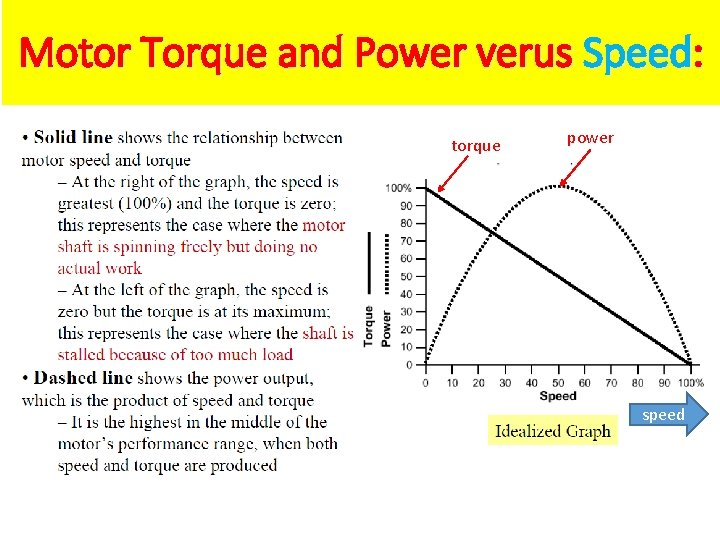Motor Torque and Power verus Speed: torque power speed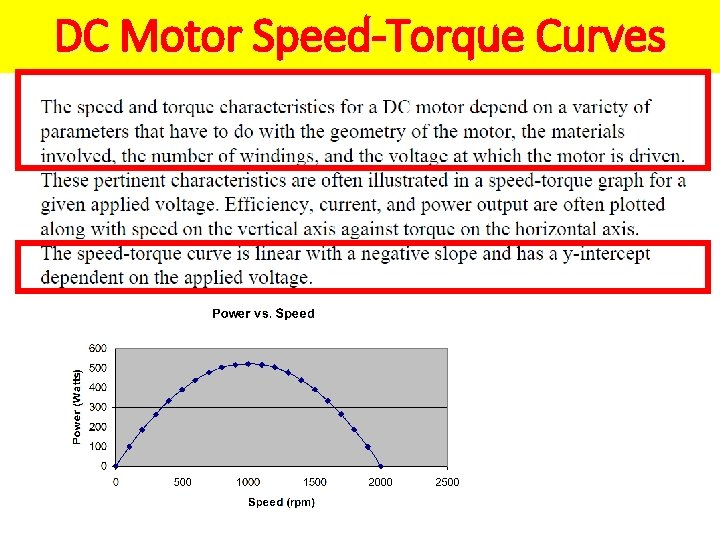DC Motor Speed-Torque Curves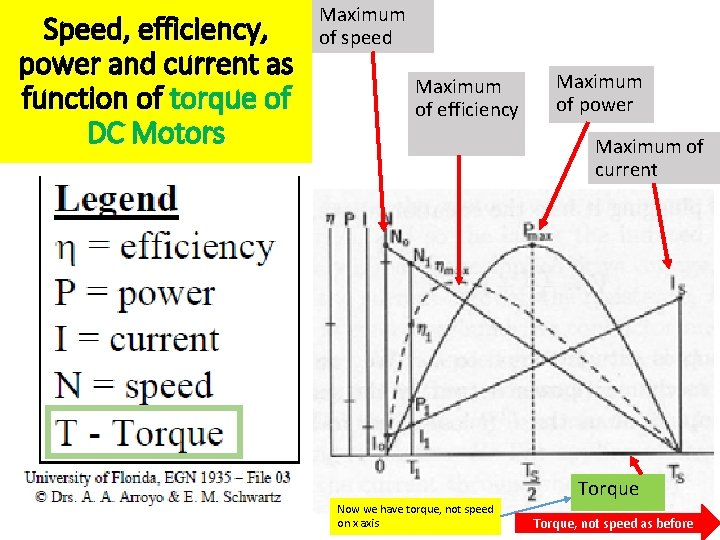Speed, efficiency, power and current as function of torque of DC Motors Maximum of speed Maximum of efficiency Maximum of power Maximum of current Torque Now we have torque, not speed on x axis Torque, not speed as before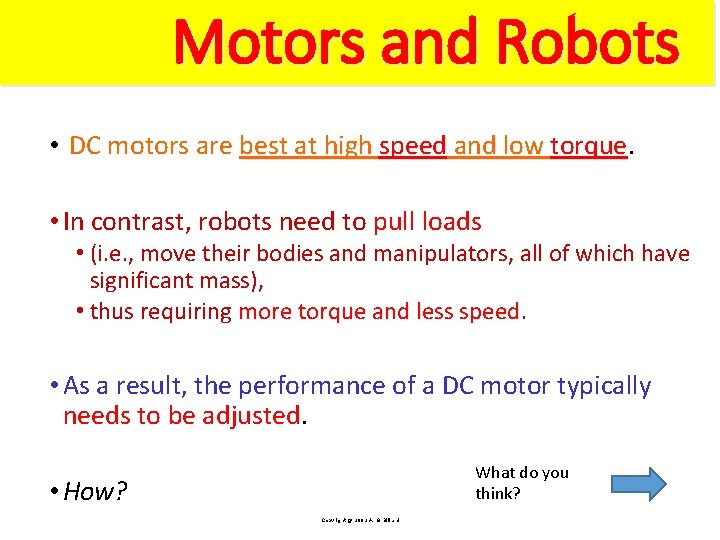Motors and Robots • DC motors are best at high speed and low torque • In contrast, robots need to pull loads • (i. e. , move their bodies and manipulators, all of which have significant mass), • thus requiring more torque and less speed • As a result, the performance of a DC motor typically needs to be adjusted. What do you think? • How? Copyright @ 2002 A. G. BillardDC Motor Speed. Torque Curves with gears Larger gear rotates slower but has more torque R 1 1, T 1 R 2 Shaft of motor 2, T 2 • The current increases linearly with torque. Current is independent of applied voltage. Maximum of speed Max voltage This is what we get from the motor Smaller current for smaller torque This is what we get from the same motor geared down with coefficient G G=R 1/R 2 smaller voltage Speed decreased G times Torque increased G times Smaller voltage, smaller speed for the same torque Torque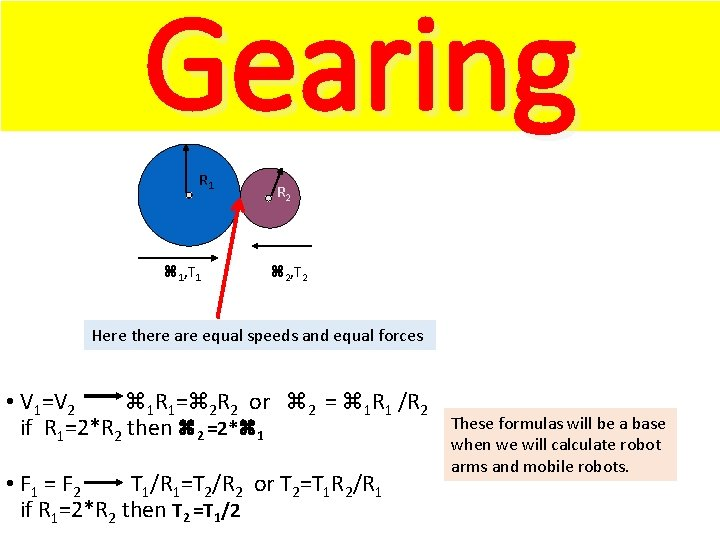Gearing R 1 1, T 1 R 2 2, T 2 Here there are equal speeds and equal forces • V 1=V 2 1 R 1= 2 R 2 or 2 = 1 R 1 /R 2 if R 1=2*R 2 then 2 =2* 1 • F 1 = F 2 T 1/R 1=T 2/R 2 or T 2=T 1 R 2/R 1 if R 1=2*R 2 then T 2 =T 1/2 These formulas will be a base when we will calculate robot arms and mobile robots.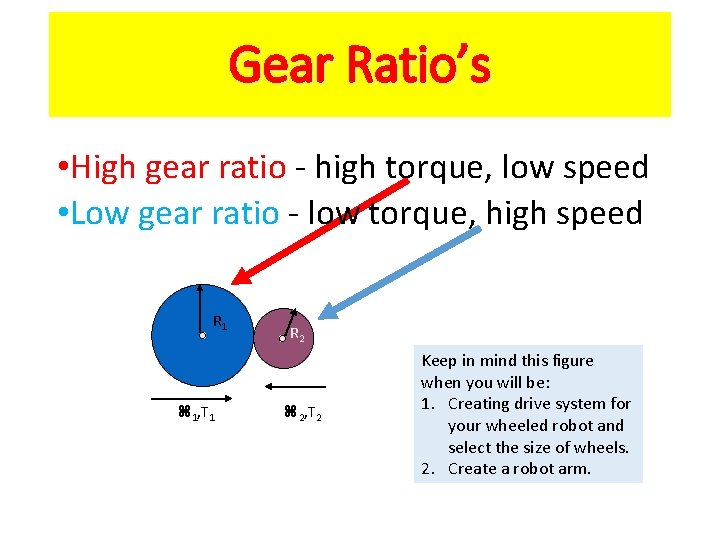Gear Ratio’s • High gear ratio - high torque, low speed • Low gear ratio - low torque, high speed R 1 1, T 1 R 2 2, T 2 Keep in mind this figure when you will be: 1. Creating drive system for your wheeled robot and select the size of wheels. 2. Create a robot arm.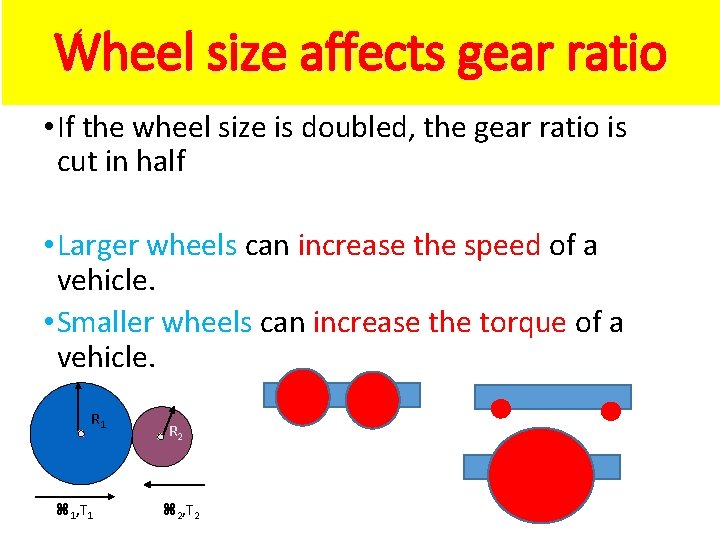Wheel size affects gear ratio • If the wheel size is doubled, the gear ratio is cut in half • Larger wheels can increase the speed of a vehicle. • Smaller wheels can increase the torque of a vehicle. R 1 1, T 1 R 2 2, T 2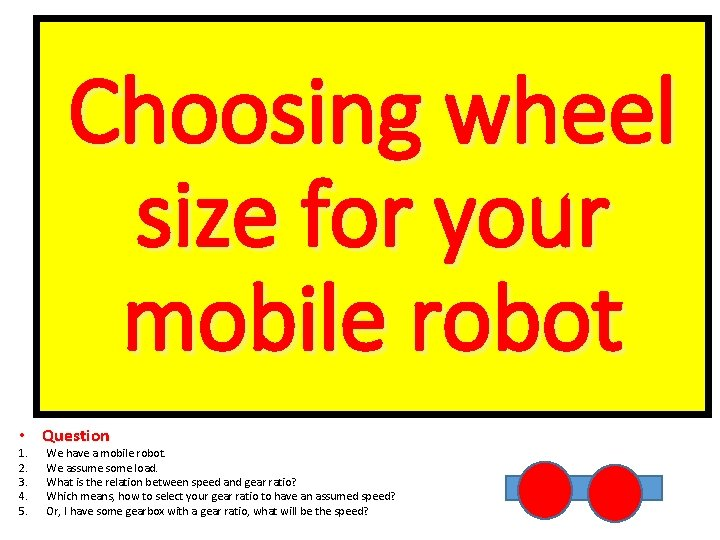Choosing wheel size for your mobile robot • Question 1. 2. 3. 4. 5. We have a mobile robot. We assume some load. What is the relation between speed and gear ratio? Which means, how to select your gear ratio to have an assumed speed? Or, I have some gearbox with a gear ratio, what will be the speed?Choosing Gear ratios Which gearing will give us a faster vehicle if our load is 9 Newtonmeters? No gearing, speed is 20 radians per second 1. Consider no gearing 9 N-m speed Torque four times higher 2. Consider 4: 1 gearing R 1 Motor 1, T 1 shaft 4: 1 gearing, speed is 42 radians per second R 2 2, T 2 Maximum speed four times smallerChoosing Gear ratios What is there is smaller load? Which gearing will give us a faster vehicle if our load is 5 Newton-meters? No gearing, 100 radians per second 5 N-m Conclusion You must know the load and the curve of your motor when you design your mobile robot for speed. 4: 1 gearing, 46 radians per secondChoosing wheel size for your mobile robot • Question 1. 2. 3. 4. 5. We have a mobile robot. We assume some load. What is the relation between speed and gear ratio? Which means, how to select your gear ratio to have an assumed speed? Or, I have some gearbox with a gear ratio, what will be the speed?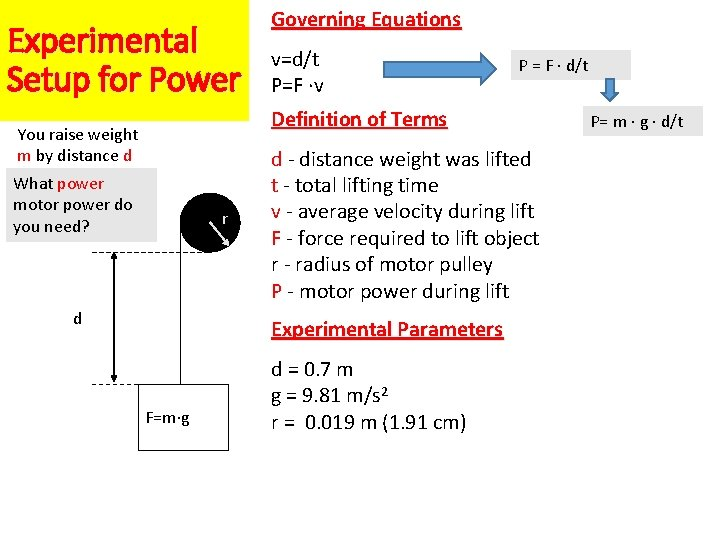Experimental Setup for Power Governing Equations v=d/t P=F ·v P = F · d/t Definition of Terms You raise weight m by distance d What power motor power do you need? r d d - distance weight was lifted t - total lifting time v - average velocity during lift F - force required to lift object r - radius of motor pulley P - motor power during lift Experimental Parameters F=m·g d = 0. 7 m g = 9. 81 m/s 2 r = 0. 019 m (1. 91 cm) P= m · g · d/tSpeed, vs Current, vs Torque, vs Power Governing Equations v=d/t P=F ·v P = F · d/t P= m · g · d/t You raise weight m by distance d Practical problems What power motor power do you need? r d F=m·g 1. Knowing m, g, d, t you want to calculate power. 2. Knowing power and some other parameters, you want to find best combination. 3. Many practical problems can be formulated that relate power, speed, torque, efficiency, current, voltage and gears. 4. You can use some Excel calculator or write a GA algorithm to optimize your problem. 5. Formulating the problem is your creative task. 6. Solving the problem is either assuming and verifying or using some kind of more advanced optimization.Motor Selection EstimatesMotor Selection Estimates 1. You have a motor. 2. You are designing a mobile robot. You know the tasks of this robot, ramps, speeds, loads. 3. Is my motor good enough? This is the power that my application requires From torque calculated for your design This is the power that my motor gives These data you find experimentally or in catalog for your motorMotor Selection Estimates (cont) 1 • Using Torque-Speed Curve to select the motor with sufficient power for your task. 2 Angular velocity Power curve current 3 torque 4 Now you know what is the value of maximum power that you need. 1. Can your motor deliver this power? 2. Good motor with this power may be expensive. 3. May be you need to have to cheap motors in parallel?Motor Selection Comments: design a drive train. 5 • Do not be too optimistic, assume low efficiency. • Have conservative estimates on drops in drive efficiency!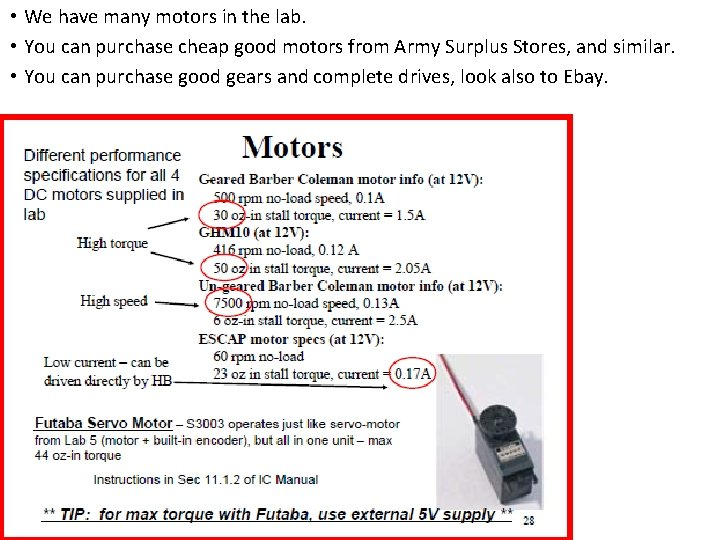• We have many motors in the lab. • You can purchase cheap good motors from Army Surplus Stores, and similar. • You can purchase good gears and complete drives, look also to Ebay.Sources • Copyright @ 2002 A. G. Billard • Zachery L Olson